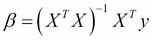# Multiple regression with gradient descent

When we ran multiple linear regression in Chapter 3, Correlation, we used the normal equation and matrices to quickly arrive at the coefficients for a multiple linear regression model. The normal equation is repeated as follows:The normal equation uses matrix algebra to very quickly and efficiently arrive at the least squares estimates. Where all data fits in memory, this is a very convenient and concise equation. Where the data exceeds the memory available to a single machine however, the calculation becomes unwieldy. The reason for this is matrix inversion. The calculation of is not something that can ...

Get Clojure for Data Science now with the O’Reilly learning platform.

O’Reilly members experience live online training, plus books, videos, and digital content from nearly 200 publishers.